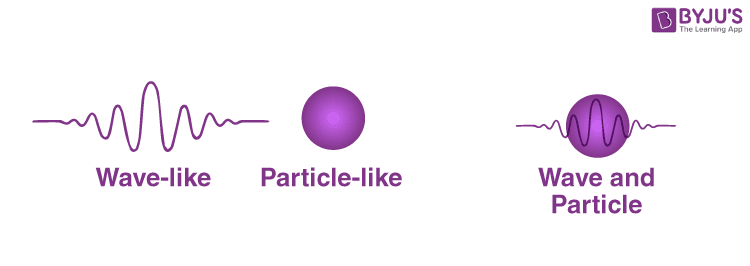Checkout JEE MAINS 2022 Question Paper Analysis : Checkout JEE MAINS 2022 Question Paper Analysis :

# Quantum Physics

We will study Quantum Physics and Classical physics. Newton’s laws of motion can explain the behaviour of macroscopic objects or objects that are at a scale of human interaction and experience, even including astronomical objects. But classical physics cannot explain the behaviour of macroscopic objects or objects that are at a scale of an atom.

### Dual Behaviour of Matter:

This is mainly because the behaviour of macroscopic objects is practically particle in nature, they do have a wave nature, but it is negligible because of their huge masses. In contrast, on the other hand, the atomic level particles have very little mass, and hence both particle and wave nature is prevalent in them. This dual behaviour of displaying both particle and wave nature is known as the dual behaviour of matter, and for every particle, the particle nature comes from its mass, and the wave nature comes from its matter-wave defined by the De-Broglie relationship, which is given by,

λ =

$$\begin{array}{l} \frac {h}{mv}\end{array}$$

Where,

λ = wavelength of the matter

h = plank’s constant

m = mass of the matter

v = velocity of matterClassical Physics hasn’t been able to explain the dual behaviour of a matter and Heisenberg’s uncertainty principle. The position and momentum of a sub-atomic particle can be calculated simultaneously with some degree of inaccuracy. Hence, there was a need for a new theory that could explain the behaviour of atomic and sub-atomic particles.

So this led to the birth of quantum physics. It is a branch of science that explains the physical phenomenon by microscopic and atomic approaches and considers the dual behaviour of matter. It is theoretical physics, and it specifies the laws of motion that microscopic objects obey. When quantum mechanics is applied to macroscopic objects (for which wave-like properties are insignificant) the results are the same as those from classical mechanics.

## Watch the video to see the standing wave in action.Stay tuned with BYJU’S for more such interesting articles. Also, register to “BYJU’S – The Learning App” for loads of interactive, engaging Physics-related videos and an unlimited academic assist.

## Frequently Asked Questions – FAQs

### What is meant by dual behaviour of matter?

Dual behaviour of matter indicates that the particle displays both particle as well as wave nature.

### What is the formula of De-Broglie relationship?

De-Broglie relationship is given by

$$\begin{array}{l}\lambda =\frac{h}{mv}\end{array}$$

### Was classical Physics successful in explaining the dual behaviour of a matter?

Classical Physics was not successful in explaining the dual behaviour of a matter.

### What is quantum physics?

Quantum physics is the branch of science that explains the physical phenomenon by microscopic and atomic approaches and considers the dual behaviour of matter.

### According to quantum physics, what is the formula to calculate the dipole moment potential?

As per quantum physics, dipole moment potential is given by U=-μB=-μzB.
Test your knowledge on Quantum Physics